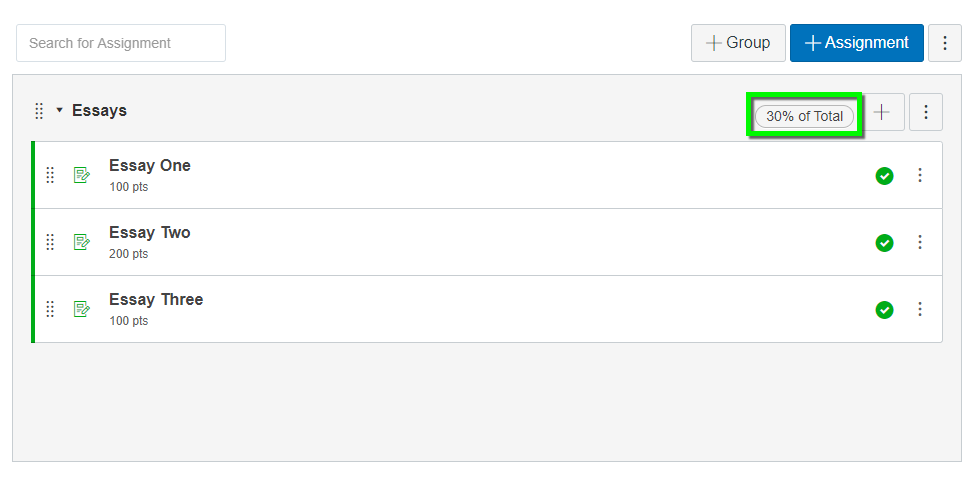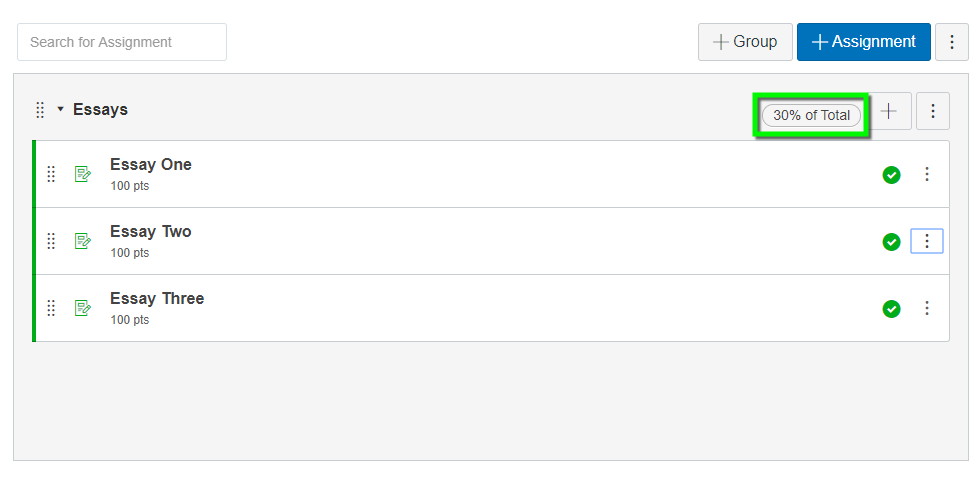# Assignment Groups

## How Do I Use Assignment Groups in Canvas?

In Canvas, assignment groups allow you to organize your assignments into discrete groups. For example, you may want to group all the essay assignments in your course within one assignment group titled ‘Essays’.

Assignment groups allow you to leverage a weighted grade scheme in your Canvas course. A weighted grade scheme allows you to distribute percentages of a student’s final grade across these assignment groups. Following our previous example, you may want the Essays group to account for 30% of the final grade.

By default, Grade weighting is turned off for all Canvas courses. To turn on Grade weighting, please follow Canvas’ tutorial for weighting final grades based on Assignment Groups.

### How do grade weights get distributed across an Assignment Group?

In Canvas, the weight of an assignment group is distributed proportionally across the assignments inside it. Because of this, assignments worth different points will be weighted differently if they are in the same assignment group.

Here’s an example of an assignment group in Canvas. This group is worth 30% of the total grade and contains three assignments with different point values. The table further below outlines how the assignment group weight of 30% is distributed across the three Assignments.Here, since Essay Two is out of 200 points, it will count two times more than Essays One and Three.

Assignment Point Value Proportion Weight (% of grade)
Essay One 100 100/400 total points .25 * 30% = 7.5%
Essay Two 200 200/400 total points .50 * 30% = 15%
Essay Three 100 100/400 total points .25 * 30% = 7.5%

***assignment group weight distribution for assignments with differing point totals.

If you want all assignments within a given assignment group to be worth the same weight, you have two options:

1. Make all assignments inside an assignment group worth the same number of points, as shown below.***In this scenario, we have changed Essay Two’s point value from 200 to 100 points. Since all point values in this assignment Group are the same, each Assignment will be worth the same, 10% in this case.

2. Create new assignment groups for any outlier assignments if you cannot or do not want to change their point value.***In this scenario, we have not changed Essay Two’s point values, so we will need to put it in its own assignment group worth 10% and change the first assignment group to be worth 20% so that all Essays are now worth 10% of the total grade.